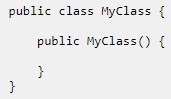# Java ConstructorsConstructors are special kinds of methods which have the following properties:

• Constructors don’t have a return type
• Constructors have the same name as the class
• Constructors are used to initialize data members
• Constructors can have arguments
• Constructor definition is optional

### Default constructors

If a constructor is not provided, the compiler uses the no-argument constructor of the superclass. If the superclass does not have a no-argument constructor, the compiler would complain. Many classes don’t have s superclass. For these classes, the class “Object” is the superclass and it provides a no-argument constructor. Not providing a constructor is bad programming and you should get in the habit of always providing constructors.

### Passing parameters to constructors

In the example on the previous page, the following constructor was provided. It assigns predetermined values to data members.

``````Calc() {
this.operand1 = 1;
this.operand2 = 2;
this.result = 0;
}``````

A constructor can accept parameters from the caller. Following is a rewrite of the previous page’s example with a constructor that accepts parameters.

``````public class Calc {
int operand1;
int operand2;
int result;

Calc(int op1, int op2) {
this.operand1 = op1;
this.operand2 = op2;
this.result = 0;
}

void calculate() {
this.result = this.operand1 + this.operand2;
}

int getResult() {
return this.result;
}
}

public class testCalc {
public static void main(String[] args) {
Calc c = new Calc(2,3);
c.calculate();
System.out.println(c.getResult());
}
}``````

When the object is instantiated, the values 2 and 3 are passed to the calculator. The constructor accepts two int values. These values are then assigned to class variables operand1 and operand2. With this change in the code, testCalc can now send any two integers and get a sum. However, a new object needs to be created for every sum. This example was only to show how to pass parameters to constructors. Don’t use this logic in your real-world programs i.e. objects creation cost memory and processing time, so never create unnecessary objects.